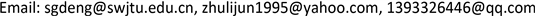﻿ 差分方程xn+1 =xn2+ xn-12解的渐近性质 On the Asymptotic Behavior of xn+1 =xn2+ xn-12

Vol.06 No.07(2017), Article ID:22550,4 pages
10.12677/AAM.2017.67107

On the Asymptotic Behavior of ${x}_{n+1}={x}_{n}^{2}+{x}_{n-1}^{2}$

Shaogao Deng1, Lijun Zhu2, Yue Chao1

1School of Mathematics, Southwest Jiaotong University, Chengdu Sichuan

2School of Mathematics and Information science, North Minzu University, Yinchuan NingxiaReceived: Oct. 8th, 2017; accepted: Oct. 24th, 2017; published: Oct. 31st, 2017ABSTRACT

This paper considers a nonlinear difference equation ${x}_{n+1}={x}_{n}^{2}+{x}_{n-1}^{2}$ with the initial values ${x}_{0},{x}_{1}\in \left(0,\infty \right)$ . The sufficient conditions under which the solutions converge to zero or diverge to infinity have been obtained.

Keywords:Difference Equation, Equilibrium Point, Asymptotically Stable1西南交通大学，数学学院，四川 成都

2北方民族大学，数学与信息科学学院，宁夏 银川1. 引言

${x}_{n+1}={x}_{n}^{2}+{x}_{n-1}^{2}$ (1.1)

${x}_{n+1}=\text{ƒ}\left({x}_{n},{x}_{n-1},\cdots ,{x}_{n-k}\right),n\in \left\{k,k+1,\cdots \right\}$ (1.2)

$\beta$ 是方程(1.2)的平衡点，令

${p}_{i}={\stackrel{˙}{f}}_{{v}_{i}}\left(\beta ,\beta ,\cdots ,\beta \right),\text{}i\in N\left(0,k\right)$

${y}_{n+1}={p}_{0}{y}_{n}+{p}_{1}{y}_{n-1}+\cdots +{p}_{k}{y}_{n-k}$ (1.3)

${\lambda }^{k+1}-{p}_{0}{\lambda }^{k}-{p}_{1}{\lambda }^{k-1}-\cdots -{p}_{k}=0$ (1.4)

${\lambda }_{1}={\lambda }_{2}=0,$

$\lambda =\left(1+\sqrt{5}\right)/2>1$

2. 主要结果

${\mathrm{lim}}_{n\to \infty }{x}_{n}=0$ .

${x}_{3}={x}_{2}^{2}+{x}_{1}^{2}<{0.5}^{2}+{0.5}^{2}=0.5$

$r=2\mathrm{max}\left\{{x}_{2},{x}_{3}\right\}$ ，则 $r<1,{x}_{2}\le 0.5r,{x}_{3}\le 0.5r$

${x}_{5}={x}_{4}^{2}+{x}_{3}^{2}\le {\left(0.5r\right)}^{2}+{\left(0.5r\right)}^{2}=0.5{r}^{2}$

${x}_{2k}\le 0.5{r}^{k},{x}_{2k+1}\le 0.5{r}^{k},k\ge 1$ ，则

${x}_{2\left(k+1\right)}={x}_{2k+1}^{2}+{x}_{2k}^{2}\le {\left(0.5{r}^{k}\right)}^{2}+{\left(0.5{r}^{k}\right)}^{2}=0.5{r}^{2k}\le 0.5{r}^{k+1}\le 0.5{r}^{k}$ ,

${x}_{2\left(k+1\right)+1}={x}_{2\left(k+1\right)}^{2}+{x}_{2k+1}^{2}\le {\left(0.5{r}^{k}\right)}^{2}+{\left(0.5{r}^{k}\right)}^{2}=0.5{r}^{2k}\le 0.5{r}^{k+1}$ .

${\mathrm{lim}}_{n\to \infty }{x}_{n}=\infty$

${x}_{3}={x}_{2}^{2}+{x}_{1}^{2}>{0.5}^{2}+{0.5}^{2}=0.5$ ,

$r=2\mathrm{min}\left\{{x}_{2},{x}_{3}\right\}$

$r>1,{x}_{2}\ge 0.5r,{x}_{3}\ge 0.5r$

${x}_{2\left(k+1\right)}={x}_{2k+1}^{2}+{x}_{2k}^{2}\ge {\left(0.5{r}^{k}\right)}^{2}+{\left(0.5{r}^{k}\right)}^{2}=0.5{r}^{2k}\ge 0.5{r}^{k+1}\ge 0.5{r}^{k}$ ,

${x}_{2\left(k+1\right)+1}={x}_{2\left(k+1\right)}^{2}+{x}_{2k+1}^{2}\ge {\left(0.5{r}^{k}\right)}^{2}+{\left(0.5{r}^{k}\right)}^{2}\ge 0.5{r}^{k+1}$ .

$\forall n\in N$$n\ge 1$${x}_{2n}\ge 0.5{r}^{n}$${x}_{\left(2n+1\right)}\ge 0.5{r}^{n}$

${x}_{1}={x}_{1}\left({x}_{0}\right),\left(0\le {x}_{0}\le c\right)$ ,

${x}_{1}$ 是关于 ${x}_{0}$ 单调递减的和经过点 $\left(0.5,0.5\right)$ ，其中c是某个常数。但要具体地求出c的值是不容易的，若要确定这条边界曲线那就更复杂了。

${x}_{1}=0$ ，则 ${x}_{2}={x}_{1}^{2}+{x}_{0}^{2}={x}_{0}^{2}$

${x}_{3}={x}_{2}^{2}+{x}_{1}^{2}={x}_{0}^{4}$ ;

${x}_{4}={x}_{3}^{2}+{x}_{2}^{2}={x}_{0}^{8}+{x}_{0}^{4}<1$ ;

${x}_{2}={x}_{1}^{2}+{x}_{0}^{2}={x}_{1}^{2}$ ;

${x}_{3}={x}_{2}^{2}+{x}_{1}^{2}={x}_{1}^{4}+{x}_{1}^{2}<1$ ;

On the Asymptotic Behavior of xn+1 =xn2+ xn-12[J]. 应用数学进展, 2017, 06(07): 892-895. http://dx.doi.org/10.12677/AAM.2017.67107

1. 1. Amleh, A.M., Grove, E.A., Ladas, G. and Georgiou, D.A. (1999) On the Recursive Sequence . Journal of Mathe-matical Analysis and Applications, 233, 790-798. https://doi.org/10.1006/jmaa.1999.6346

2. 2. Stevic, S. (2009) Boundedness Cha-racter of a Class of Difference Equations. Nonlinear Analysis, 70, 839-848. https://doi.org/10.1016/j.na.2008.01.014

3. 3. 徐胜荣, 王希超, 周营营. 一类差分方程的稳定性研究[J]. 山东农业大学学报(自然科学版), 2013, 44(4): 624-629.

4. 4. 尤严. 一类非线性二阶差分方程Robin问题多个正解的存在性[J]. 吉林大学学报(理学版), 2017, 55(2): 257-261.

5. 5. Elaydi, S. (2005) An Introduction to Difference Equations. 3rd Edition, Springer-Verlag, Ber-lin.